#### Time optimality

Interesting interpretations of the minimum principle exist for the case of optimizing the time to reach the goal [424,903]. In this case,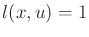in (15.26), and the cost term can be ignored. For the remaining portion, let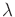be defined as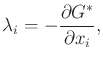(15.40)

instead of using (15.25). In this case, the Hamiltonian can be expressed as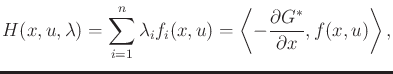(15.41)

which is an inner product between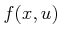and the negative gradient of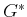. Using (15.40), the Hamiltonian should be maximized instead of minimized (this is equivalent to Pontryagin's original formulation ). An inner product of two vectors increases as their directions become closer to parallel. Optimizing (15.41) amounts to selectingso that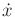is as close as possible to the direction of steepest descent of. This is nicely interpreted by considering how the boundary of the reachable set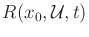propagates through. By definition, the points on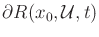must correspond to time-optimal trajectories. Furthermore,can be interpreted as a propagating wavefront that is perpendicular to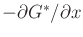. The minimum principle simply indicates thatshould be chosen so thatpoints into the propagating boundary, as close to being orthogonal as possible .

Steven M LaValle 2020-08-14